Math Calculators, Lessons and Formulas

It is time to solve your math problem

mathportal.org

# Complex number calculator

The calculator does the following: extracts the square root, calculates the modulus, finds the inverse, finds conjugate and transforms complex numbers into polar form. For each operation, the solver provides a detailed step-by-step explanation.

problem

Find the modulus of

$$z = 2+i$$

solution

The polar form of $z$ is:

$$z = \sqrt{ 5 } \cdot \left ( cos ~ 27^o + i \cdot sin ~ 27^o \right)$$

explanation

The polar form of the complex number $z = a + bi$ is:

$$z = r \cdot \left(cos~\theta + i \cdot sin ~ \theta \right)$$

where, $r$ is the modulus of $z$ and $~ \theta ~$ is an argument of $z$.

The moduo for $a = 2$ and $b = 1$ is:

$$r = \sqrt{a^2 + b^2} = \cdots =\sqrt{ 5 }$$

To find argument $\theta$ we use one of the following formulas:

\begin{aligned} \theta & = \arctan \left( \frac{b}{a} \right) \text{ if } a > 0 \\ \theta & = \arctan \left( \frac{b}{a} \right) + 180^o \text{ if } a < 0 \\ \\ \theta & = 90^o \text{ if } a = 0 \text{ and } b > 0 \\ \\ \theta & = 270^o \text{ if } a = 0 \text{ and } b < 0 \end{aligned}

In this example: $a = 2$ and $b = 1$ so:

$$\theta = arctan \left( \frac{b}{a} \right)$$$$\theta = arctan \left( \frac{ 1 }{ 2 } \right)$$$$\theta = arctan \left( \frac{ 1 }{ 2 } \right)$$$$\theta \approx 27^o$$

## Report an Error !

Script name : complex-unary-operations-calculator

Form values: 2 , 1 , 1 , pol , g , Find the modulus conjugate of 2+i , ,

Comment (optional)

## Share Result

Or just copy and paste the link wherever you need it.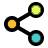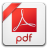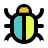Operations with complex number
five operations with a single complex number
show help ↓↓ examples ↓↓ tutorial ↓↓
$z = 4 + 6i$
$z = 2 - \frac{3}{2}i$
$z = \sqrt{2} - \sqrt{5}i$
 Modulus (Magnitude) Conjugate Inverse Roots Polar form
Find approximate solution
Hide steps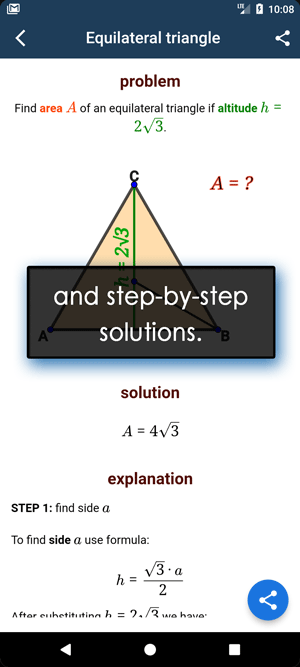working...
EXAMPLES
example 1:ex 1:
Find the complex conjugate of $z = \frac{2}{3} - 3i$.
example 2:ex 2:
Find the modulus of $z = \frac{1}{2} + \frac{3}{4}i$.
example 3:ex 3:
Find the inverse of complex number $3 - 3i$.
example 4:ex 4:
Find the polar form of complex number $z = \frac{1}{2} + 4i$.
TUTORIAL

## Operations on complex numbers

This calculator performs five operations on a single complex number.
It computes module, conjugate, inverse, roots and polar form.

### 1 : Modulus ( Magnitude )

The modulus or magnitude of a complex number ( denoted by $\color{blue}{ | z | }$ ), is the distance between the origin and that number.

If the $z = a + bi$ is a complex number than the modulus is

$$|z| = \sqrt{a^2 + b^2}$$Example 01: Find the modulus of $z = \color{blue}{6} + \color{purple}3{} i$.

In this example $\color{blue}{a = 6}$ and $\color{purple}{b = 3}$, so the modulus is:

\begin{aligned} | z | &= \sqrt{ a^2 + b^2} = \sqrt{6^2 + 3^2} = \\[1 em] &= \sqrt{36 + 9} = \sqrt{45} = \\[1 em] &= \sqrt{9 \cdot 5} = 3 \sqrt{5} \end{aligned}

### 2 : Conjugate

To find the complex conjugate of a complex number, we need to change the sign of the imaginary part. The conjugate of $z = a \color{red}{ + b}\,i$ is:

$$\overline{z} = a \color{red}{ - b}\,i$$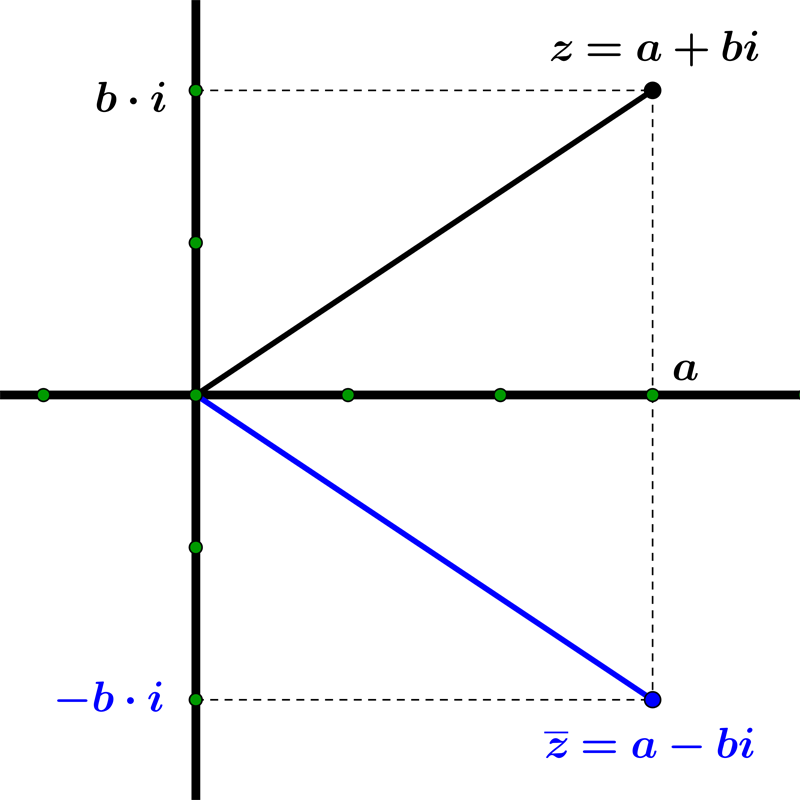Example 02: The complex conjugate of $~ z = 3 \color{blue}{+} 4i ~$ is $~ \overline{z} = 3 \color{red}{-} 4i$.

Example 03: The conjugate of $~ z = - 4i ~$ is $~ \overline{z} = 4i$.

Example 04: The conjugate of $~ z = 15 ~$ is $~ \overline{z} = 15 ~$, too.

### 4 : Inverse

The inverse or reciprocal of a complex number $a + b\,i$ is

$$\color{blue}{ \frac{1}{a + b\,i}}$$

Here is an example of how to find the inverse.

Example 06: Find the inverse of the number $z = 4 + 3i$

\begin{aligned} \frac{1}{z} &= \frac{1}{4+3i} = \frac{1}{4+3i} \cdot \frac{4-3i}{4-3i} = \\[1 em] &= \frac{4-3i}{(4+3i)(4-3i)} = \frac{4-3i}{4^2 - (3i)^2} = \\[1 em] &= \frac{4-3i}{16+9} = \frac{4-3i}{25} = \frac{4}{25} - \frac{3}{25} i \end{aligned}

### 3 : Polar Form

The polar form of a complex number $z = a + i\,b$ is given as $z = |z| ( \cos \alpha + i \sin \alpha)$.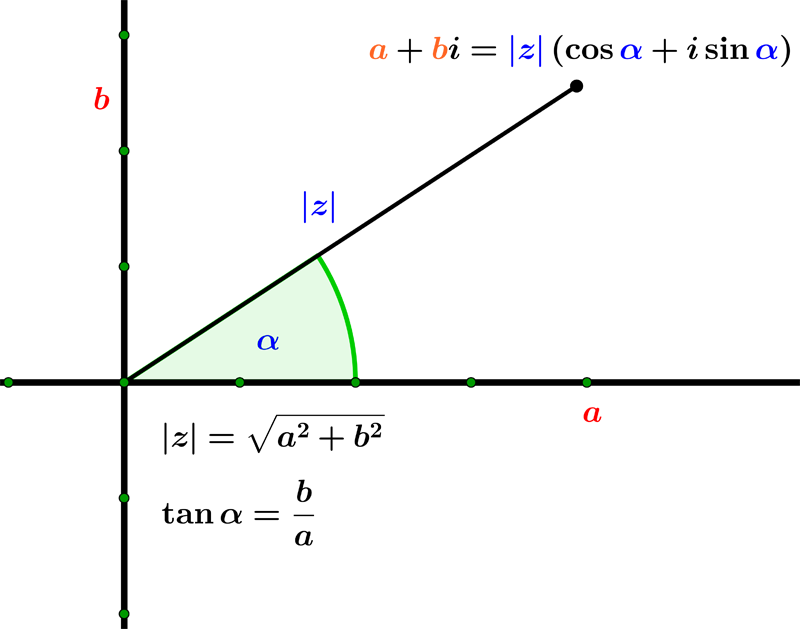Example 05: Express the complex number $z = 2 + i$ in polar form.

To find a polar form, we need to calculate $|z|$ and $\alpha$ using formulas in the above image.

$$|z| = \sqrt{2^2 + 1^2} = \sqrt{5}$$ $$\tan \alpha = \dfrac{b}{a} = \dfrac{1}{2} \implies \alpha = \tan^{-1}\left(\dfrac{1}{2}\right) \approx 27^{o}$$

So, the polar form is:

$$z = \sqrt{5} \left( \cos 27^{o} + i \sin27^{o} \right)$$
Search our database of more than 200 calculators